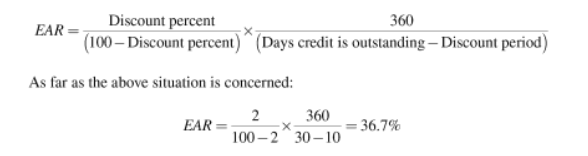Can anyone explain the cost of trade credit formula?

Suppose that the credit terms are 2/10, net 30: you get a 2% discount if you pay within 10 days, otherwise, the total amount is due in 30 days. You can think of the price as 98% of the total, and the interest at 2% of the total; thus, your effective interest rate for 20 days (= 30 – 10) is 2.0408% (= 2% / 98%). You compound that to get the annual rate:

cost of trade credit = (1 + (discount % / (1 – discount %))365/days past discount – 1

In this example,

cost of trade credit = (1 + (2% / 98%))365/20 – 1

= 44.59%

Cool! Thanks a lot. Still, not very intuitive. (How would effective intr rate for 20 days be 2/98; that 98 price was valid only for 10 days; not after that)

I am not applying my brainThink of paying the total on day 30 this way: you pay 98% on day 10 (taking advantage of the discount), then borrow that 98% back for 20 days (so your cash flow on day 10 is zero); after 20 days you have to pay 100%, or 2% more, on a loan of 98%.

1 Like

S2000magician be God _/_

Discount Percent/100 - Discount percent X 365/Days Credit is outstanding - Discount period = Cost of Not Taking the Discount.

Cost Of Trade Credit is called Cost of Not Taking the Discount.

For Example: Let’s say that your company is offered terms of trade of 2/10, net 30.This means that the supplier will offer you a 2 percent discount if you pay your bill in 10 days.Now, we have to imagine a scenario where your company is not able to take that 2 percent discount.What is this going to cost u ?

Using our example:

2/100 -2 X 365/30 - 10 = 37.2%

This formula is incorrect. It’s not compounding the cost; the formula that CFA Institute has in Corporate Finance compounds the cost.

I have a question, in your example of 2/10, net 30

Do you get the “days past” discount by multiplying 2*10 or by 30-10? My guess would be 30-10 but I’m not sure.

cost of trade credit = (1 + (discount % / (1 – discount %))365/days past discount – 1

In this example,

cost of trade credit = (1 + (2% / 98%))365/20 – 1

= 44.59%

30 − 10

Why would you think it is 2*10? Where did you get 2 from? If you got 2 from 2/10, then you need to understand that 2 means 2% if repaid within the initial 10 days. Can’t multiply % with days to get days. That’s like adding apples to oranges and calling the result apples. BTW in this instance apples + oranges doesn’t equal squash :P.

Thanks, I’m starting to get this.

Good to hear.